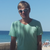# QlikView App Dev

Discussion Board for collaboration related to QlikView App Development.

Announcements
Skip the ticket, Chat with Qlik Support instead for instant assistance.
cancel
Showing results for
Did you mean:Master II

## Macro Problem

I have a vba macro with this line:

Range("A1").Resize(UBound(x)) = Application.Transpose(x)

when i run the macro from excel everything works fine.

Now i try to run the macro from QV module with this adaption:

objWorkbook.Sheets(1).Range("A1").Resize(UBound(x)) = objExcel .Transpose(x)

but it throws an error: Typenkonflikt: 'objExcel.Transpose'

inside the Excel i use this code and it works perfectly:

```Sub Test()
Dim IE As Object
Set IE = CreateObject("InternetExplorer.Application")
With IE
.Visible = False
.Navigate "http://test.com"
Do Until .Busy Or .ReadyState = 4: DoEvents: Loop
Dim i As Integer
msgbox("1")
x = .document.body.innertext
x = Replace(x, Chr(10), Chr(13))
x = Split(x, Chr(13))
Range("A1").Resize(UBound(x)) = Application.Transpose(x)
.Quit
End With
End Sub

```

this I use in Qlikview Module

```Sub Test
Dim x
Set objExcel = CreateObject("Excel.Application")
objExcel.Visible = True
Set IE = CreateObject("InternetExplorer.Application")
With IE
.Visible = False
.Navigate "http://test.com"
Do Until .Busy Or .ReadyState = 4: DoEvents: Loop
msgbox("1")
x = .document.body.innertext
x = Replace(x, Chr(10), Chr(13))
x = Split(x, Chr(13))
objWorkbook.Sheets(1).Range("A1").Resize(UBound(x)) = objExcel.Transpose(x)
.Quit
End With
objExcel.Quit
End Sub

```

is anybody able to tell me the äquivalent in QV module

I have attached the working xlsm file

and also the not working qvw

1 Solution

Accepted SolutionsMaster II
Author

this is what finally worked for me:

Sub Test

Dim x

Dim dteWait

Set objExcel = CreateObject("Excel.Application")

objExcel.Visible = False

Set IE = CreateObject("InternetExplorer.Application")

With IE

.Visible = True

.Navigate "http://cryptoindexes.com/volatility"

Do Until .Busy Or .ReadyState = 4: DoEvents: Loop

Do Until (Now() > dteWait)

Loop

x = .document.body.innertext

x = Replace(x, Chr(10), Chr(13))

Set WshShell = CreateObject("WScript.Shell")

Set oExec = WshShell.Exec("clip")

Set oIn = oExec.stdIn

oIn.WriteLine x

oIn.Close

objExcel.Range("A1").PasteSpecial

End With

objWorkbook.Save

objExcel.Quit

IE.Quit

End Sub

5 RepliesMaster II
Author

any ideas on this?MVP & Luminary

Not yet tested with your examples but could it be that your array is too big for transpose which could be handle max. 65536 values? If yes, you will need another approach with looping through the array and it might be in general not a really slower way, see: Array Transponieren - - - - - - - - - - - - Office-Loesung.de

- MarcusMaster II
Author

Hi Marcus,

But why is the vba solution working with transpose and the vbs solution not?

shouldn´t there be the limitation of 65536 values for both solutions using  transpose?MVP & Luminary

My hint was initiated from the error-message "Typenkonflikt" and the first search hinted on the object-size. Now I looked again and there are further possibilities for such errors if the array contained NULL or the value-lenghts > 255. Again both arrays should be the same when created in the above way - but I'm not sure if VBA and VBS are behaving identically in such cases - maybe one ignored this occurence and the other failed. It's maybe not the next step to do but you could read (and write in excel) the values of your array to see if you hit the max. number of values or of the value-lenghts or NULL.

Another point could be the call of Application.Transpose which is outdated and might be replaced with something like:

Application.WorksheetFunction.Transpose(myarray)

arrays - vba tranpose type mismatch error - Stack Overflow

https://bettersolutions.com/vba/arrays/transposing.htm

-  MarcusMaster II
Author

this is what finally worked for me:

Sub Test

Dim x

Dim dteWait

Set objExcel = CreateObject("Excel.Application")

objExcel.Visible = False

Set IE = CreateObject("InternetExplorer.Application")

With IE

.Visible = True

.Navigate "http://cryptoindexes.com/volatility"

Do Until .Busy Or .ReadyState = 4: DoEvents: Loop

Do Until (Now() > dteWait)

Loop

x = .document.body.innertext

x = Replace(x, Chr(10), Chr(13))

Set WshShell = CreateObject("WScript.Shell")

Set oExec = WshShell.Exec("clip")

Set oIn = oExec.stdIn

oIn.WriteLine x

oIn.Close

objExcel.Range("A1").PasteSpecial

End With

objWorkbook.Save

objExcel.Quit

IE.Quit

End SubCommunity Browser